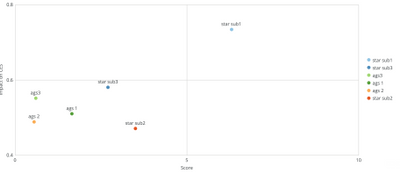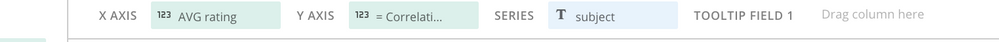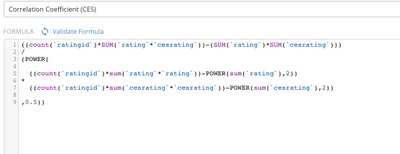# Adding background image to embedded cards

Hey all,

I'm having a bit of trouble trying to figure out how to add a background image to a card that is powered primarily by beast mode calcuations. I've endlessly searched the forums and have not yet been able to come up with a solution. What I have is a matrix with 4 quadrants that need to be color-coded and labeled. Here is what I've tried so far and why these options are insufficient:

1. App Design Studio - This card displays correlation coefficients which can only be calculated via beast mode. As far as I have researched, the Design Studio will not allow table caclulations to be the main source for the colums.

2. Custom svg files - I pretty much run into the same issue as the point made above.

3. Adding html code into the beast mode - Doing this would effectively take away our correlation coefficients, so this option is out.

Anybody have a solution or experience with handling something like this?

EDIT:  I'm also adding that this card needs to be embdedded, which elimates the option of adding a background image to a story board.

Tagged:

• For anybody who may have been following this topic,

I've figured out how to calculate the Pearsons correlation coefficient within the SQL dataflow. It was quite simple actually. All I had to do was select all attributes and add in this calculation I originally had in BeastMode:

(((count(`ratingid`) * SUM((`x` * `y`))) - (SUM(`x`) * SUM(`y`))) / POWER((((count(`ratingid`) * sum((`x` * `x`))) - POWER(sum(`x`),2)) * ((count(`ratingid`) * sum((`y` * `y`))) - POWER(sum(`y`),2))),0.5)) AS "Correlation"

It worked out for me and I got the same exact results as when I ran the calculation within beastmode. With "Correlation" as a table column, we should now be able to create a card within the design studio that does not require a table calculation.

Feel free to message me if you come accross this and need more of an explanation.

•CoachCould you attach some screenshots of what you currently have and where you are trying to get to?

“There is a superhero in all of us, we just need the courage to put on the cape.” -Superman
• Thanks for your reply. I've attached some screenshots and provided a bit of explanation into what we're trying to do.This is what we currently have, however we want to assign each quadrant a separate filled color. This helps our users understand a little better what each quadrant means.^^ Here is the layout for our card axis settings. The correlation coefficient (at our current stage) can only be calculated via beast mode since we have thousands upon thousands of segments for this data. As far as I know, this is incompatible with cards created in design studio.This is the underlaying formula for our correlation coefficient. I've searched the Dojo for ways to accomplish this in ETL but haven't found anything that would satisfy our needs.

• For anybody who may have been following this topic,

I've figured out how to calculate the Pearsons correlation coefficient within the SQL dataflow. It was quite simple actually. All I had to do was select all attributes and add in this calculation I originally had in BeastMode:

(((count(`ratingid`) * SUM((`x` * `y`))) - (SUM(`x`) * SUM(`y`))) / POWER((((count(`ratingid`) * sum((`x` * `x`))) - POWER(sum(`x`),2)) * ((count(`ratingid`) * sum((`y` * `y`))) - POWER(sum(`y`),2))),0.5)) AS "Correlation"

It worked out for me and I got the same exact results as when I ran the calculation within beastmode. With "Correlation" as a table column, we should now be able to create a card within the design studio that does not require a table calculation.

Feel free to message me if you come accross this and need more of an explanation.

•CoachJust be careful, when you calculate this within the dataflow, it will no longer respond to any filters you are applying to the card.  For example, if you calculate the corelation of two events occuring in the same calendar year in a dataflow.  Then the corelation number will not recalculate if you change your card to look at a 2 year period.

“There is a superhero in all of us, we just need the courage to put on the cape.” -Superman
• This was one of the tradeoffs we were willing to deal with until Domo adds support for table calculations within cards created via design studio. It seems like a pretty basic function to me though. Hopefully the feature will be added soon.

This discussion has been closed.